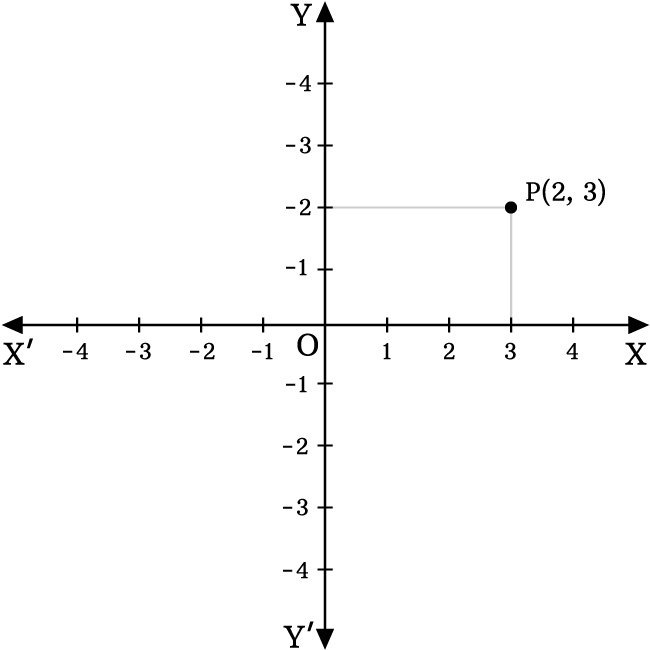# Two dimensional space

A coordinate system in which the exact position of a point is determined with two numerical values, is called the two dimensional space.

## IntroductionIn Euclidean geometry, two perpendicular number-lines are intersected at their middle point for splitting the whole space into four equal parts. The number lines on the perpendicular lines are used as base line to measure the distance of every point in two perpendicular directions from their bisection point.

This geometric setting helps us to determinate the exact position of every point by two numerical values in the plane and this Cartesian system is called the two-dimensional space.

The two dimensional space is also called by the following three names.

1. Two dimensional Cartesian coordinate system.
2. Bi-dimensional Cartesian coordinate system.
3. Bi-dimensional space.

### Basics

There are four fundamental concepts to start learning the two dimensional Cartesian coordinate system.

Latest Math Topics
Jun 26, 2023
Jun 23, 2023

Latest Math Problems
Jul 01, 2023
Jun 25, 2023
###### Math Questions

The math problems with solutions to learn how to solve a problem.

Learn solutions

Practice now

###### Math Videos

The math videos tutorials with visual graphics to learn every concept.

Watch now

###### Subscribe us

Get the latest math updates from the Math Doubts by subscribing us.